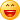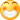# 时空各维可变系多线矢量子数形矢算物理学(6)哈！
本节由创新建立的时空矢量运算，具体导出了，各种可能的，各类正、反，时空[多线矢]，及其相互作用、结合、演变，的，特性、规律。

1. 把时空6维，统一的力，误认为。时轴分量可忽略的3维空间的静磁力，硬造出个所谓“磁单极”
2. 量子色动力学，因缺少4维时空多线矢算，不能区分时空各

我国物理学家，杨振宁和李政道在共同研究，电磁驰豫过程，的对称性时，第一次发现“弱力作用下，宇称对称性不守恒”，并由吴健雄用实验证实。就已经发现：实为12维的弱力，与3维的，引力、6维矢量、的电磁力，有显著差别，对全面认识4种相互作用力，起着关键的重要作用，引起国际科学界极大的重视。后来，又有物理学家，在分析基本粒子演变时，仍然仅按3维、4维矢量的对称性分析，发现“实为12维的，强力作用下，对称性‘不守恒’” 而错误地得出，所谓“对称性的‘自发破缺’”。
3.杨振宁的“规范场”理论的重要性，在于：把3维空间和4维时空矢量，拉格朗日量的规范对称性，推广用于实为12维的，强力、弱力，而促进了量子色动力学“标准模型”的发展。但因，没有4维的矢算,不能导出高于4维的各类多线矢。所谓“夸克模型”，就把6维的粒子，当作2个3维夸克的粒子、把12维的粒子，当作3个4维夸克的粒子，彼此禁闭成团。其实，既无单个的夸克，任何粒子又不可能在时空禁闭成团，因而，所谓“夸克模型”根本不能成立。
必须尽快，弥补、纠正、创新、发展，才能，全面、正确，地认识、掌握、运用，改造、发展，各类量子，服务于人类不断增长的物质和文化需求！
有重要的基础理论意义和广泛的实用作用。
热诚欢迎网友们，特别是有关专家 ，积极参与，讨论、创新、发展！6.各维时空矢量的矢量运算表达式

6.1.各维时空矢量的加、减，分别是由，各相同分量相加、减。

A(4)叉乘B(4)

={iA0+Aj[j],j=13求和}

(+-){iB0+Bj[j],j=13求和}

={i(A0(+-)B0)+(Aj(+-)Bj)[j],j=13求和}

={iC0+Cj[j],j=13求和}

=4维时空[线矢]C(4)

6.2.各类时空矢量的叉乘，相应的正、反，多线矢、标量的产生，及其有关数据

24维时空[1线矢]的叉乘

A(4)叉乘B(4)

={iA0+Aj[j],j=13求和}

={i(A0Bj-AjB0)[0j]+(AkBl-AlBk)[kl],jkl=123循环求和}

={iC0j[0j]+Ckl[kl],jkl=123循环求和}

=6维时空[2线矢]=C(6)

6维时空[2线矢]叉乘4维时空[1线矢]

A(6)叉乘B(4)

={iA0j[0j]+Akl[kl],jkl=123循环求和}

={i(A0kBl-A0lBk[0kl]+(AjkBl-AlBjk)[jkl],jkl=123循环求和}

={iC0kl[0kl]+Cjkl[jkl],jkl=123循环求和}

=C(4)=4维时空[3线矢]

={iC0*[0*]+Cj*[j*],j=13求和}

=4维时空反[1线矢]=4维时空[1*线矢]=C(4)[1*]

44维时空[1线矢]的叉乘

=4维时空[3线矢]叉乘4维时空[1线矢]

=4维时空[1*线矢]叉乘4维时空[1线矢]

=4维时空[3线矢]叉乘4维时空[1线矢]

=4维时空矢量4维行列式[标量]

26维时空[2线矢]的叉乘

{iC0j[0j]+Ckl[kl],jkl=123循环求和}

=15维时空[2,2线矢]=E(15)[2,2]

={iE0j,kl[0j,kl]-E0k,0l[0k,0l]-E0l,0k[0l,0k]

+Ekl,lj[kl,lj]+Elj,kl[lj,kl],jkl=123循环求和}

15维时空[2,2线矢]叉乘6维时空[2线矢]

A(15)[2,2]叉乘B(6)

={iA0j,kl[0j,kl]-A0k,0l[0k,0l]-A0l,0k[0l,0k]

+Akl,lj[kl,lj]+Alj,kl[lj,kl],jkl=123循环求和}

={iA0j,kl[0j,kl]-A0k,0l[0k,0l]-A0l,0k[0l,0k]

+Akl,lj[kl,lj]+Alj,kl[lj,kl],jkl=123循环求和}

=6维时空[2,2,2线矢]=C(6)[2,2,2]

={iC0j,kl,lj[0j,kl,lj]

-C0k,0l,lj[0k,0l,lj]-C0l,0k,jk[0l,0k,jk]

+Ckl,lj,jk[kl,lj,jk]+Clj,kl,jk[kl,lj,jk]

,jkl=123循环求和}

=6维时空反[2线矢]

={iC0j*[0j*]+Ckl*[kl*],jkl=123循环求和}=C(6)[2*]

15维时空[2,2线矢]叉乘4维时空[1线矢]

A(15)[2,2]叉乘B(4)

={iA0j,kl[0j,kl]-A0k,0l[0k,0l]-A0l,0k[0l,0k]

+Akl,lj[kl,lj]+Alj,kl[lj,kl],jkl=123循环求和}

=12维时空[2,2,1线矢]=C(12)[2,2.1]

={iC0,kl,lj[0,kl,lj]+Cj,kl,lj[j,kl,lj],jkl=123循环求和}

(注意:A(15)[2,2]点乘B(4)=12维时空反[2,2,1线矢]

=C(12)[2,2.1*])

215维时空[2,2线矢]的叉乘

=46维时空[2线矢]的叉乘

=46维时空[2*线矢]的叉乘

=6维时空矢量4维行列式[标量]

6.3.各类时空矢量的点乘，相应的正、反，多线矢、标量的产生

4维时空矢量，的点乘：

4维时空[1线矢]点乘4维时空[1线矢]

{iA0+Aj[j],j=13求和}

={-A0B0+AjBj,j=13求和}[标量]

6维时空[2线矢]点乘4维时空[1线矢]

{iA0j[0j]+Akl[kl],jkl=123循环求和}

={iC0+Cj[j]+Ck[k]-Cl],jkl=123循环求和}

={iC0+Cj[j],j=13求和}=C(4)

4维时空[3线矢]4维时空[1线矢]

=4维时空[1*线矢]点乘4维时空[1线矢]

{iA0kl[0kl]+Ajkl[jkl],jkl=123循环求和}

={iC0k[0k]-iC0l[0l]+iC0j[0j]+Ckl[kl]-Cjk[jk]

+Clj[lj],jkl=123循环求和}

={iC0j[0j]+Ckl[kl],jkl=123循环求和}=C(6)

15维时空[2,2线矢]4维时空[1线矢]

{iA0j,kl[0j,kl]-A0k,0l[0k,0l]-A0l,0k[0l,0k]

+Akl,lj[kl,lj]+Alj,kl[lj,kl],jkl=123循环求和}

=12维时空反[2,2,1线矢]=C(12*)[2,2.1*]

={iC0,kl,lj*[0,kl,lj*]+Cj,kl,lj*[j,kl,lj*],jkl=123循环求和}

(注意:A(15)[2,2]叉乘B(4)=12维时空[2,2,1线矢]

=C(12)[2,2.1*])=C(12)[2,2.1])

(未完待续)

http://wap.sciencenet.cn/blog-226-1288822.html

## 全部精选博文导读

GMT+8, 2021-10-21 03:57